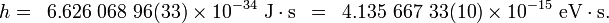# Planck's constant

The Planck constant (denoted h) is a natural constant named after Max Planck, one of the founders of quantum theory. Its value is approximately h = 6.63 × 10-34 J s. A closely-related quantity is the reduced Planck constant (also known as Dirac's constant and denoted ħ, pronounced "h-bar").

Planck discovered in 1901 his constant in his study of black-body radiation and formulated a law ("Plancks's law") for the density of black-body radiation as a function of temperature. Four years later Einstein rederived Planck's law by assuming that electromagnetic radiation consists of parcels of energy hν, where ν is the frequency of the radiation. These energy parcels are now called photons. The Planck constant is ubiquitous in quantum mechanics. For instance, it appears in the Schrödinger equation. Its small size is the main reason that quantum effects are only noticeable for microscopic particles such as electrons and nuclei.

## Units, value and symbols

The Planck constant has dimensions of energy multiplied by time, which are also the dimensions of action. In SI units, the Planck constant is expressed in joule-seconds. The dimensions may also be written as momentum times distance (N·m·s), which are also the dimensions of angular momentum.

The value of the Planck constant is:$h =\,\,\, 6.626\ 068\ 96(33) \times10^{-34}\ \mbox{J}\cdot\mbox{s} \,\,\, = \,\,\, 4.135\ 667\ 33(10) \times10^{-15}\ \mbox{eV}\cdot\mbox{s}.$

The two digits between the parentheses denote the standard uncertainty in the last two digits of the value.

The value of the Dirac constant is:$\hbar\ \equiv \frac{h}{2\pi} = \,\,\, 1.054\ 571\ 628(53)\times10^{-34}\ \mbox{J}\cdot\mbox{s} \,\,\, = \,\,\, 6.582\ 118\ 99(16) \times10^{-16}\ \mbox{eV}\cdot\mbox{s}.$

## Footnotes

1. Planck constant. 2006 CODATA recommended values. NIST. Retrieved on 2007-08-08.
2. Planck constant over 2 pi. 2006 CODATA recommended values. NIST. Retrieved on 2007-08-08.Some content on this page may previously have appeared on Citizendium.